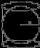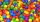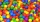# Division + sphere - math problems

#### Number of problems found: 8

• Inscribed sphereHow many percent of the cube volume takes the sphere inscribed into it?
• Volume of sphereHow many times does the volume of a sphere increase if its radius increases 2 ×?
• Equilateral cylinderA sphere is inserted into the rotating equilateral cylinder (touching the bases and the shell). Prove that the cylinder has both a volume and a surface half larger than an inscribed sphere.
• Cube in a sphereThe cube is inscribed in a sphere with a volume 7253 cm3. Determine the length of the edges of a cube.
• Thousand ballsWe have to create a thousand balls from a sphere with a diameter of 1 m. What will be their radius?
• Clay ballsHow many clay balls with a radius of 1 cm can be made from a ball of clay with a radius of 8 cm?
• CannonballsOf the three cannonballs with a diameter of 16 cm, which landed in the castle courtyard during the battle, the castle blacksmith cast balls with a diameter of 10 cm, which fit into the cannons placed on the walls. How many cannonballs did the blacksmith c
• The cylindrical containerThe cylindrical container has a base area of 300 cm3 and a height of 10 cm. It is 90% filled with water. We gradually insert metal balls into the water, each with a volume of 20 cm3. After inserting how many balls for the first time does water flow over

We apologize, but in this category are not a lot of examples.
Do you have an interesting mathematical word problem that you can't solve it? Submit a math problem, and we can try to solve it.

We will send a solution to your e-mail address. Solved examples are also published here. Please enter the e-mail correctly and check whether you don't have a full mailbox.

Please do not submit problems from current active competitions such as Mathematical Olympiad, correspondence seminars etc...

Division Problems. Sphere Problems.